Feature Extraction Techniques in Associate with Opposition Based Whale Optimization Algorithm

Feature Extraction Techniques in Associate with Opposition Based Whale Optimization Algorithm

Gottumukkala HimaBinduChinta Anuradha Patnala S.R. Chandra Murty

Department of Computer Science & Engineering, College of Engineering & Technology, Acharya Nagarjuna University, Guntur, Andhra Pradesh, India

Department of Computer Science & Engineering, V. R. Siddhartha Engineering College, Vijayawada, Andhra Pradesh, India

Corresponding Author Email:
ghimabindu19@gmail.com
Page:
403-410
|
DOI:
https://doi.org/10.18280/isi.240407
13 April 2019
|
Accepted:
27 July 2019
|
Published:
10 October 2019
| Citation

OPEN ACCESS

Abstract:

Near-duplicate video (NDV) detection is an important issue of copyright protection. However, the traditional detection methods are very imprecise and complex. To solve the problem, this paper introduces the opposition-based solution generation strategy into the conventional whale optimization algorithm (OWOA), creating a novel NDV detection method called the OWOA. The author detailed how to use the hybrid method to extract different types of features, ranging from color, shape to texture, and compared the OWOA with traditional feature extraction methods through experiments. The comparison shows that the OWOA achieved the optimal performance in DNV detection. The research findings can greatly assist regulatory authorities in monitoring and detecting edited contents.

Keywords:

near-duplicate video (NDV) detection, digital rights management, feature extraction, optimization techniques, the opposition-based whale optimization algorithm (OWOA)

1. Introduction

Semantic depiction of long videos is basic in the solicitations like audiovisual statistics recovery, spontaneous pouring, pictorial damaged persons self-care, audiovisual observation, senior and children attention . A video is frequently viewed as a fair-minded record of events, and since it is generally troublesome not to confide in the proof of our own eyes, digital videos present probably the most compelling documentary proof conceivable, both inside and outside the court . If each frame in a video is treated as an autonomous image, there are just such a large number of images to process proficiently .

Video can be anticipated as an arrangement of images named frames, appeared over a period of time. As such, the altering recognition techniques delivered for image forensics [4-8] could be connected at frame level. The characteristics trajectories encrypt image belongings, to be specific color, quality, and form. The comparison amid two images is figured as a function of the distance between their feature vectors . Various inquiries have been done on the textural examination of images by proposing distinctive feature extraction methods. The extracted features similar histogram, DWT, GLCM, and GLRLM are analyzed independently . Textural features can likewise be extracted from the spectral domains, for example, the frequency domain, the wavelet domain, and the Gabor domain . Utilizing this framework a user is empowered with a facility to give a transferring platform for videos where while transferring a video watermark utilizing DWT is connected and Keyframe extraction is done . In this procedure, which will be referred to as WOA, the bubble-net stalking method of whales implanted in the WOA is made use of to enhance the characteristics and look for the finest subsection of structures . Whale Optimization Algorithm (WOA) is a hunt and development procedure , freshly generated. It is a carefully worked-out imitation of the drive and conduct of humpback whales in their hunt for nutrition and necessities. By relying upon average feature value of entirely the frames in the segments, keyframes are to be resolute. For matching propose, a keyframe is utilized and remaining frames are utilized to distinguish segment location .

2. Literature Review

Soumya Prakash Rana et al.  2019, had anticipated an image was endless by a single feature. Along these lines, the examination keeps an eye on that point for content built image recovery (CBIR) by linking variable color and form structures with non-variable quality characteristics. At long last, a theory test was done to set up the consequence of the suggested effort which initiates assessed exactness and reminiscence values are valid and recognized for the all image database.

Hardi M. Mohammed et al.  2019, had suggested a precise and meta-investigation survey of WOA was led to assist scientists with using it in various areas or hybridize it with other collective algorithms. Next, WOA exhibitions are displayed to solve various issues. The survey’s outcomes demonstrate that WOA performs superior to anything other normal algorithms regarding meeting haste and harmonizing amongst assessment and utilization.

Sitara and Mehtre  2018 had proposed videos are adequate as proof in the court of law, given its credibility and integrity are logically approved. In that paper, they propose inter-frame fake discovery approaches using meddle hints from Spatio-temporal and compacted dominions. Experimental outcomes demonstrate that they have effectively noticed edge scuffling with positive correctness charges. They have well versed enhanced correctness on fake discovery in edge inclusion, edge removal, and edge repetition.

Sowmya et al.  2018, had expected a method to notice inter edge and intra edge audiovisual fake exploiting content-built sign. The planned STTFR procedure supposes to approve audiovisual uprightness via the formation of a 128 tad message abridgment from the input audiovisual of a modifiable span that will be strange to that audiovisual and drives around as an impression. The skill of the sign won't authorize any distinct/material to replicate the unique audiovisual as the sign was produced by linking three-dimensional and chronological impressions in an arranged and organized method.

Yang et al.  2016, had intended replication of chosen edges from an audiovisual to additional part in a similar audiovisual was a standout amongst the most extensively predictable methods of audiovisual counterfeit. The trial results exhibit that our algorithm gives detection accuracy that was higher than the past algorithms, and it has an exceptional performance in terms of time effectiveness.

3. Proposed Methodology

The important purpose of this investigation effort is to recognize NDV detection and non-NDV detection. The process initiates from training which incorporates video to frames and feature extractions. Feature extractions methods involved in this process are DWT (12), shape (1), color (1), and texture (99) on collective consider as 113 features. This research anticipates by utilizing OWOA technique for predicting optimal features to recognize NDV detection and non-NDV detection. During testing, when a system received query video the preliminary step is to convert it to frames and feature extraction techniques applied and then consider the optimal features for Euclidian distance comparison to recognize NDV detection and non-NDV detection. This research utilized 50 videos for training and 15 videos for testing. The subsequent Figure 1 displays the block-Figure for complete suggested method.

1.jpg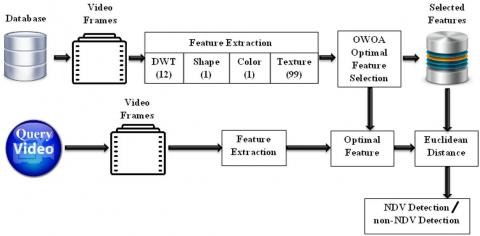Figure 1. Block diagram for overall proposed methodology

3.1 Video to frame conversion

To play out the work further video cannot legitimately use for distinguishing proof procedure so convert video into image frames. After that frames convert into blocks then to retrieve accurate predicting rate feature extraction place an important role.

3.2 Feature extraction

The images consequent to frames and the feature extracted by a technique for regulating DWT, Shape, Color, Texture (GLCM and GLRLM) in the feature extraction procedure.

3.2.1 Distinct wavelet transmute (DWT)

In article, wavelets change the image into a sequence of wavelets that can be set down more capably than pixel blocks. Wavelets have coarse boundaries. They are able to condense images greater by positioning the sound pixels. Image includes pixels that are prearranged in two dimensional matrixes; each pixel signifies the ordinal correspondence of image strength. In three-dimensional area adjoining pixel values are intensely connected and thereafter laid off. So as to squeeze images, these dismissals predominant amid pixels ought to be given out with. DWT processor alters the three-dimensional area pixels into incidence area data that are signified in numerous sub-bands, signifying different period and occurrence points. It is categorized as Receive Convert and Daubechies wavelet. This feature incorporates Coefficient of Approximation (CA), Coefficient of Horizontal (CH), Coefficient of vertical (CV), and Coefficient of Diagonal (CD) which processed through mean, standard deviation and variance on collective consider as 12 features.

Mean formula

$Mean=\frac{Sum\,\,of\,\,All\,\,Data\,\,Po\operatorname{int}s}{Number\,\,of\,\,Data\,\,Po\operatorname{int}s}$       (1)

Standard deviation

For an arbitrary inconstant trajectory A containing N scalar interpretations, the normal deviancy is dissimilar as

$S=\sqrt{\frac{1}{N-1}\sum\limits_{i=1}^{N}{{{\left| {{A}_{i-\mu }} \right|}^{2}}}}$      (2)

where, µ is the mean of A:

$\mu =\frac{1}{N}\sum\limits_{i=1}^{N}{{{A}_{i}}}$        (3)

The normal deviancy is the square root of the difference. Certain descriptions of normal deviancy made use of a standardization feature of N as an alternative of N-1, which you can recognize by locating w to 1.

Difference

For an arbitrary inconstant trajectory A containing N scalar interpretations, the difference is different as

$V=\frac{1}{N-1}\sum\limits_{i=1}^{N}{{{\left| {{A}_{i-\mu }} \right|}^{2}}}$      (4)

where, µ is the mean of A:

$\mu =\frac{1}{N}\sum\limits_{i=1}^{N}{{{A}_{i}}}$        (5)

A few descriptions of difference use a standardization feature of N fairly than N-1, which can be designated by locating w to 1. In both the circumstances, the mean is believed to have the normal standardization feature N.

3.2.2 Shape feature extraction

The form is important cause of evidence which is utilized for item appreciation. Without shape visual content item can't recognize appropriately. Image is inadequately deprived of identifying form. The two substances cannot have identical shape yet by means of numerous procedures we can identify related form efficaciously. In this numerical morphology technique is used and it gives an approach to managing the treatment of digital images which relies upon shape. Appropriately used, Mathematical morphological operations tend to extract their fundamental shape credits and to crash irrelevancies.

3.2.3 Color feature removal

Color expanse signifies the color in the form of strength value. We can display, imagine and create the color by means of color expanse method. There are exclusive color feature removal methods.

(1) Color histogram

The color histogram represents the image from alternate point of view. The appearance in which color boxes of regularity circulation are signified by color histogram and it totals the pixels which are comparative and preserve it. Color histogram examines all the arithmetical color regularity in an image. The variation happened in the conversion, revolution, and position of vision problems are clarified by color histogram and further it emphases on distinct shares of an image. The computation of local color histogram is simple and it is impermeable to minor variations in the image so for indexing and retrieval of image database it is basic.

3.2.4 Texture feature extraction

Texture contains basic information about the major game plan of the exterior that is hazes, greeneries, blocks, cloth and so on. Texture feature also portrays the somatic arrangement of surface. There are various procedures of texture feature extraction. Here includes GLCM and GLRLM; GLCM includes four rotation like 0º, 45º, 90º and 135º for 22 features and GLRLM consists of 11 on collective consider as 99 features.

(1) Grey Level Co-occurrence Matrix (GLCM)

A GLCM consistently implies a matrix in which the amount of rows and columns are proportionate to the number of combine of gray levels with value G, in the image. Each feature suggests the texture uniformity and non-uniformity, similarity, dissimilarity and various parameters. On the moldy scope that the image is fundamentally sufficiently altered, by then the similarity is increasingly indisputable and if the image is not in any way altered, at that time the similarity is equivalent to one.

$Autocorrelation=\,\,\,\,\sum\limits_{v}{\sum\limits_{u}{uvp(u,v)}}\,$         (6)

$Contrast\,=\,\,\,\,\sum\limits_{v}{\sum\limits_{u}{{{(u-v)}^{2}}}}\,p(u,v)$      (7)

$Correlation1=\,\,\,\,\sum\limits_{v}{\sum\limits_{u}{\frac{(u-{{m}_{u}})(v-{{m}_{v}})p(u,v)}{{{\sigma }_{u}}{{\sigma }_{v}}}}}\,$ (8)

$Correlation2=\,\,\,\,\sum\limits_{v}{\sum\limits_{u}{\frac{(uvp(u,v)-{{m}_{u}}{{m}_{v}})}{{{\sigma }_{u}}{{\sigma }_{v}}}}}\,$  (9)

$Cluster\Pr o\min ence=\,\,\,\,\sum\limits_{u}{\sum\limits_{v}{{{((u-{{m}_{u}})+(v-{{m}_{v}}))}^{4}}p(u,v)}}$ (10)

$ClusterShade=\,\,\,\,\sum\limits_{u}{\sum\limits_{v}{{{((u-{{m}_{u}})+(v-{{m}_{v}}))}^{3}}p(u,v)}}$     (11)

$Dissimilarity=\sum\limits_{u}{\sum\limits_{v}{|u-v|p(u,v)}}\,\,$         (12)

$Energy=\sum\limits_{u}{\sum\limits_{v}{p{{(u,v)}^{2}}}}\,\,$                 (13)

$Entrop{{y}^{{}}}Huv\,=\,\,\,-\,\sum\limits_{u,v}{p\,(u,v)\log (p\,(u,v))}\,$        (14)

$Homogeneity1=\,\,\,\,\sum\limits_{v}{\sum\limits_{u}{\frac{p(u,v)}{1+|u-v|}}}\,$          (15)

$Homogeneity2=\,\,\,\,\sum\limits_{v}{\sum\limits_{u}{\frac{p(u,v)}{1+{{(u-v)}^{2}}}}}\,$        (16)

$Maximum\Pr obability=\max (\max (p(u,v)))$     (17)

$SumOfSquares\,=\,\,\,\,\sum\limits_{v}{\sum\limits_{u}{(u-mean(}}p(u,v)){{)}^{2}}p(u,v)$(18)

$SumAverag{{e}^{{}}}=\,\,\,\,\sum\nolimits_{m=2}^{2{{N}_{g}}}{{{p}_{u+v}}(m)}$            (19)

where, $p_{u+v}(m)$ is the probability of P(u,v) summing to u+v

${{p}_{u+v}}(k)=\sum\limits_{m}{\sum\limits_{n}{p(u,v)}}$ for i+j =k with k=0,12,…,2(N-1)

${{p}_{u-v}}(k)=\sum\limits_{m}{\sum\limits_{n}{p(u,v)}}$ for |i-j|=k with k=0,12,…,(N-1)

$SumVariance=\,\,\,\,\sum\nolimits_{m=2}^{2{{N}_{g}}}{{{(m-{{f}_{8}})}^{2}}{{p}_{u+v}}(m)}$  (20)

$SumEntrop{{y}^{{}}}{{f}_{8}}=\,\,-\sum\nolimits_{m=2}^{2{{N}_{g}}}{{{p}_{u+v}}{{(m)}^{{}}}\log \{{{p}_{u+v}}(m)\}}$(21)

$DifferenceVariance=\,\,\,\,\sum\nolimits_{m=0}^{{{N}_{g}}-1}{{{m}^{2}}{{p}_{u-v}}(m)}$ (22)

$DifferenceEntropy=\,\,\,\,-\sum\nolimits_{m=0}^{{{N}_{g}}-1}{{{p}_{u-v}}{{(m)}^{{}}}\log \{{{p}_{u-yv}}(m)\}}$ (23)

$InverseDifferenc{{e}^{{}}}INV=\,\,\,\,\sum\limits_{v}{\sum\limits_{u}{\frac{p(u,v)}{|u-v{{|}^{k}}}}}\,$      (24)

$InverseDifferenceNomalised=\,\,\,\,\sum\limits_{v}{\sum\limits_{u}{\frac{p(u,v)}{1+{{\left( \frac{|u-v|}{N} \right)}^{{}}}}}}\,$   (25)

$InverseDifferenceMomentNomalised=\,\,\,\,\sum\limits_{v}{\sum\limits_{u}{\frac{p(u,v)}{1+{{\left( \frac{u-v}{N} \right)}^{2}}}}}\,$ (26)

$InformationMeasureOfCorrelation1=\,\frac{Huv-Huv1}{\max \{Hu,Hv\}}$   (27)

$InformationMeasureOfCorrelation2=\,{{(1-\exp [-2(Huv2-Huv)])}^{\frac{1}{2}}}$(28)

where, Hu, Hv are the entropies of pu and pv probability density functions with m as u index and n as v index.

${{p}_{u}}(m)=\sum\limits_{n}^{{}}{p(m,n)}$  and ${{p}_{v}}(n)=\sum\limits_{m}^{{}}{p(m,n)}$         (29)

$Huv1=\,\,\,\,-\sum\limits_{m}{\sum\limits_{n}{p(m,n)\log \{{{p}_{u}}(m){{p}_{v}}(n)\}}}$           (30)

$Huv2=\,\,\,\,-\sum\limits_{m}{\sum\limits_{n}{{{p}_{u}}(m){{p}_{v}}(n)\log \{{{p}_{u}}(m){{p}_{v}}(n)\}}}$        (31)

${{m}_{u}}=\,\,\,\,\sum\limits_{v}{\sum\limits_{u}{up(u,v)}}\,$   ${{m}_{v}}=\,\,\,\,\sum\limits_{v}{\sum\limits_{u}{vp(u,v)}}\,$         (32)

${{\sigma }_{u}}=\,\,\,\,\sum\limits_{v}{\sum\limits_{u}{{{(u-{{m}_{u}})}^{2}}p(u,v)}}\,$

${{\sigma }_{v}}=\,\,\,\,\sum\limits_{v}{\sum\limits_{u}{{{(v-{{m}_{v}})}^{2}}p(u,v)}}\,$                    (33)

(2) Grey-level run-length matrix (GLRLM)

Quality structures centered on this GLRL matrix, viz.: Shot Runs Emphasis (SRE), Long Run Emphasis (LRE), Gray Level Non-uniformity (GLN), Run Length Non-uniformity (RLN), and Run Percentage (RP), Low Gray-Level Run Emphasis (LGRE), High Gray-Level Run Emphasis (HGRE), Short Run Low Gray-Level Emphasis (SRLGE), Short Run High Gray-Level Emphasis (SRHGE), Long Run Low Gray-Level Emphasis (LRLGE), Long Run High Gray-Level Emphasis (LRHGE).

Short Run Emphasis (SRE):

$SRE=\frac{1}{n}\sum\limits_{q,r}{\frac{p\,(q,r)}{{{r}^{2}}}}\,\,$     (34)

Long Run Emphasis (LRE):

$LRE=\frac{1}{n}\sum\limits_{q,r}{{{r}^{2}}*p\,(q,r)}\,\,\,\,$       (35)

Gray Level Non-uniformity (GLN):

$GLN=\frac{1}{n}{{\sum\limits_{q}{\left( \sum\limits_{r}{p\,(q,r)} \right)}}^{2}}\,\,\,\,$       (36)

Run Length Non-uniformity (RLN):

$RLN=\frac{1}{n}{{\sum\limits_{q}{\left( \sum\limits_{u}{p\,(q,r)} \right)}}^{2}}\,\,\,\,\,\,\,$      (37)

Run Percentage (RP):

$RP=\sum\limits_{q,r}{\frac{n}{p\,(q,r)*r}}\,\,$       (38)

Low Gray-Level Run Emphasis (LGRE):

$LGRE=\frac{1}{n}\sum\limits_{q,r}{\frac{p\,(q,r)}{{{q}^{2}}}}\,\,$        (39)

High Gray-Level Run Emphasis (HGRE):

$HGRE=\frac{1}{n}\sum\limits_{q,r}{p\,(q,r).\,\,{{q}^{2}}}\,\,\,\,$        (40)

Short Run Low Gray-Level Emphasis (SRLGE):

$SRLGE=\frac{1}{n}\sum\limits_{q,r}{\frac{p\,(q,r)}{{{q}^{2}}.\,{{r}^{2}}}}\,\,$   (41)

Short Run High Gray-Level Emphasis (SRHGE):

$SRHGE=\frac{1}{n}\sum\limits_{q,r}{\frac{p\,(q,r)\,.\,{{q}^{2}}}{{{r}^{2}}}}\,\,$      (42)

Long Run Low Gray-Level Emphasis (LRLGE):

$LRLGE=\frac{1}{n}\sum\limits_{q,r}{\frac{p\,(q,r)\,.\,{{r}^{2}}}{{{q}^{2}}}}\,\,$       (43)

Long Run High Gray-Level Emphasis (LRHGE):

$LRHGE=\frac{1}{n}\sum\limits_{q,r}{p(q,r)\,.\,{{q}^{2}}\,.\,{{r}^{2}}}\,\,$      (44)

3.3 Opposition based whale optimization algorithm (OWOA)

This procedure is enthused by the shooting contrivance of humpback whales in landscape and replicates the lessening surrounding, curved apprising location, and arbitrary shooting contrivances of humpback whale shells. This procedure is prepared for the associated three stages: surrounding quarry, fizzy mesh confronting, and hunt for quarry. And it labors as tracks with that opposition based solution generation strategy are used to upgrade the performance and the flowchart for OWOA is demonstrated in Figure 2.

3.3.1 Initial solution

This is the preliminary processing step in optimization techniques which incorporates solution length and number of solutions. Here, the number of solutions is ten and solution (array) length is similar to the number of features considered. Solutions hold randomly placed 0 and 1, which is an index of each employed features. The position (index) holding 1 and their corresponding features are considered for initial iteration. Opposition based solution generation is a procedure utilized to trap the solution from opposite end to build the probability of finding reasonable solution.

${{Y}_{i}}(i=1,2,....n),\,\,\,\,\,\,\,Max\_iter$      (45)

$O{{p}_{i}}=x+y-{{Y}_{i}}$       (46)

Yi ϵ i=1,2, …. n, these are randomly generated initial solution utilized to generate opposition based solution where x and y are minimum and maximum values.

2.jpg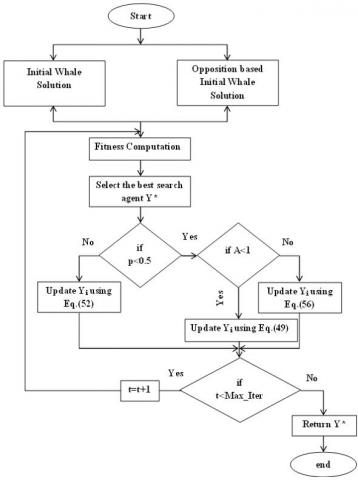Figure 2. Flowchart for OWOA

3.3.2 Fitness computation

The generated random solutions along with opposition based solution generation are evaluated through fitness computation signify below.

$Accuracy=\frac{Correctly\,\,predicted\,\,data}{Total\,no.\,of\,data}$       (47)

3.3.3 Encircling prey

For surrounding quarry, the WOA procedure receives that the present ideal resolution is the objective quarry. Further distinct whales at that point attempt to update their positions toward the optimal position. This behavior is represented by the accompanying conditions:

$D=\left| \vec{c}\cdot \vec{Y}*(t)-\vec{Y}(t) \right|$     (48)

$\vec{Y}(t+1)=\vec{Y}*(t)-\vec{A}\cdot D$     (49)

where, t signifies the present repetition, →Y(t) is the location trajectory, →Y (t) is the location trajectory of the ideal resolution developed till now, || is the complete value, and ‘.’ denotes element-by-element reproduction. →A and →C are constant trajectories and are resolute as pursues:

$\vec{A}=2\vec{a}\vec{r}-\vec{a}$    (50)

$\vec{c}=2\vec{r}$  (51)

where, →a is linearly reduced from 2 to 0 during the progression of repetitions (in both investigation and utilization stages) and →r is an arbitrary trajectory in [0, 1].

3.3.4 Bubble-net attacking method (exploitation phase)

The bubble-net conduct of humpback whales includes the lessening surrounding contrivance and curved apprising location.

(1) Lessening surrounding contrivance

This scheme is mainly proficient by lessening the approximation of device factor a. →A is an arbitrary value in the recess [−a, a]. When arbitrary values for →A are in the interval [−1, 1], the novel location of the distinct whales can be categorized anywhere amid the unique location and the present finest location. The precise demonstration is transferred by Eqns. (48) and (49).

(2) Curved apprising location

A curved equation is then done amid the location of whale and quarry to imitate the helix-shaped drive of humpback whales as pursues:

$\vec{Y}'(t+1)=\vec{D}'\cdot {{e}^{bl}}\cdot \cos \,\,\,(2\pi l)+\vec{Y}*(t)$        (52)

$\vec{D}'=\left| \vec{Y}*(t)-\vec{Y}(t) \right|$       (53)

where, b is a constant for describing the form of the logarithmic curve, l is an arbitrary numeral in [−1, 1], and is an element by-element multiplication.

At the point when humpback whales assault their prey, they change throughout interior to a lessening surrounding loop and alongside a spiral-shaped track. The WOA assume there is a 50 % possibility of gathering amid the lessening surrounding contrivance and the curved prototype to inform the location of whales in the course of development. The numerical model is as per the following:

$\vec{Y} *(t+1)=\left\{\begin{array}{ll}{\vec{Y} *(t)-\vec{A} \cdot \vec{D}} & {\text { if } p<0.5} \\ {\vec{D'} \cdot e^{b l} \cdot \cos (2 \pi l+\vec{Y} *(t)} & {\text { if } p \geq 0.5}\end{array}\right.$     (54)

3.3.5 Hunt for quarry (search stage)

Humpback whales hunt arbitrarily as per the location of each other. In the hunt for quarry or search stage, the locations of the remaining distinct whales are informed in relation to an arbitrarily chosen distinct whale.

To find out further healthier quarry, distinct whales are bound to transfer distantly from mention whales by locating |→A > 1|. WOA procedure can do global hunt by this method. The precise prototype can be conversed as:

$D=\left| \vec{c}{{\cdot }_{^{\xrightarrow[{{Y}_{ran{{d}^{(t)}}}}]{}-\vec{Y}(t)}}} \right|$        (55)

$\vec{Y}(t+1)=\xrightarrow[{{Y}_{ran{{d}^{(t)}}}}]{}-\vec{A}\cdot D$       (56)

where, Yrand is a location trajectory arbitrarily chosen from the existing whale populace.

3.4 Euclidean distance

The Euclidean distance d between two vectors X and Y is:

$d=sum\,\,(\,(x-y)\,.{{\,}^{\wedge }}2)\,.{{\,}^{\wedge }}0.5$     (57)

4. Results and Discussion

The purpose of this research objective investigate through various performance evaluations like accuracy, sensitivity, specificity, False Positive Rate (FPR), Positive Predictive Value (PPV), Negative Predictive Value (NPV), False Discovery Rate (FDR) and False Negative Rate (FNR) for employed optimization techniques such as OWOA, WOA, PSO, EA and GA. Investigation evident the incorporation of opposition strategy apparent the proficient performance of OWOA in predicting optimal features and recognizing NDV detection and non-NDV detection. The following investigation details the performance of employed techniques with respect to different standard measures, optimal features evaluate from different techniques and converging performance of employed techniques and the entire execution implement on the working platform MATLAB.

Table 1 shows the performance evaluation terminology then demonstrates the performance of employed techniques for query videos and Table 2 explained the techniques and their features count w.r.t accuracy.

Table 1. Performance of employed techniques for query videos

 Video OWOA WOA PSO GA EA All features Biking NDV detection NDV detection NDV detection NDV detection NDV detection NDV detection Billards NDV detection NDV detection Non-NDV detection Non-NDV detection Non-NDV detection NDV detection Clean And Jerk Non-NDV detection NDV detection NDV detection NDV detection NDV detection NDV detection Diving Non-NDV detection NDV detection Non-NDV detection Non-NDV detection Non-NDV detection Non-NDV detection

Table 2. Techniques and their features count w.r.t accuracy

 Particulars Count Accuracy All features 113 53.3% OWOA 60 93.3% WOA 56 80% PSO 57 73.3% GA 57 60% EA 53 66.6%

The succeeding Figure 3 demonstrates the performance of employed techniques for evaluating sensitivity. The OWOA and WOA attain 88.8 % sensitivity which is 11.1 % greater than PSO and GA, 22.2 % superior to EA and 33.3 % better performance than All features. In this graph OWOA performed superior when compared with other techniques.

The following Figure 4 illustrates the performance of employed techniques for evaluating specificity. The OWOA attains 100 % specificity which is 33.4 % greater than WOA and PSO, and 50 % better performance than EA, GA, All features. In this graph, OWOA performed superior when compared with other techniques.

The ensuing Figure 5 explains the performance of employed techniques for evaluating accuracy. The OWOA achieves 93.3 % accuracy which is 13 % greater than WOA, 20 % superior than PSO, 26.7 % better than GA, 33.3 % greater than EA and 40 % better performance than All features. In this graph OWOA performed higher when compared with other techniques.

3.jpg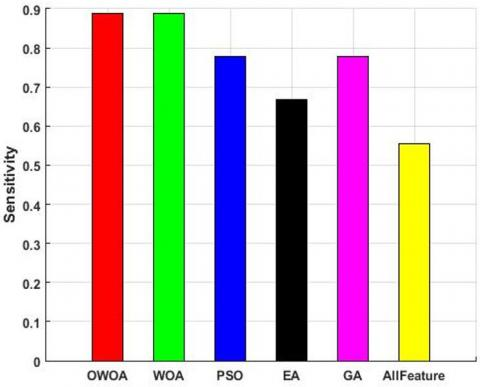Figure 3. Performance graph for Sensitivity

4.jpg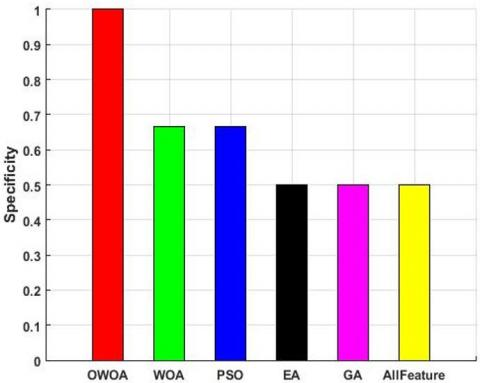Figure 4. Performance graph for Specificity

5.jpg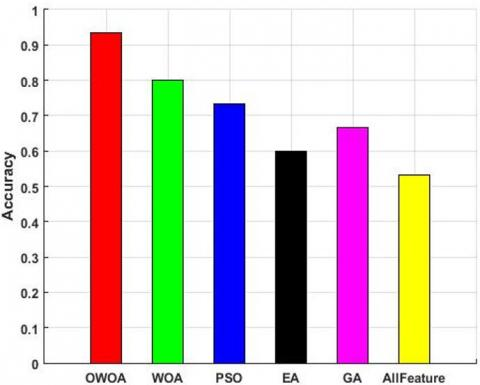Figure 5. Performance graph for accuracy

5. Conclusion

This research concludes the effectiveness of incorporating optimization techniques in the context of NDV detection with feature extraction methods. Rather than considering entire feature extraction methods for testing, this research utilizes appropriate features which reduce the complexity and enhance the overall performances. This research also utilizes opposition strategy in conventional WOA, which increases the probability to trap the best solution with respect to accuracy. Among investigated features, accuracy is a key measure which determines the actual performance of employed techniques while recognizing appropriate feature selection for NDV detection. Here, OWOA unveils 93.3 % accuracy which is 13.3 % greater than conventional WOA and 40 % superior to considering entire feature extraction process in count. This research potential accommodates and remunerates various commercial and non-commercial applications like broadcast-stream monitoring and detection of edited contents for regulatory authorities.

References

 Ding, S., Qu, S., Xi, Y., Wan, D. (2019). A long video caption generation algorithm for big video data retrieval. Future Generation Computer Systems, 93: 583-595. https://doi.org/10.1016/j.future.2018.10.054

 Singh, R.D., Aggarwal, N. (2017). Detection and localization of copy-paste forgeries in digital videos. Forensic Science International, 281: 75-91. https://doi.org/10.1016/j.forsciint.2017.10.028

 Johnston, P., Elyan, E. (2019). A review of digital video tampering: From simple editing to full synthesis. Digital Investigation, 29: 67-81. https://doi.org/10.1016/j.diin.2019.03.006

 Cozzolino, L.V.D., Poggi, G. (2014). A feature-based approach for image tampering detection and localization. IEEE International Workshop on Information Forensics and Security, pp. 149-154. https://doi.org/10.1109/wifs.2014.7084319

 Li, C., Ma, Q., Xiao, L., Li, M., Zhang, A. (2017). Image splicing detection based on Markov features in QDCT domain. Neurocomputing, 228: 29-36. https://doi.org/10.1016/j.neucom.2016.04.068

 Zhao, X., Wang, S., Li, S., Li, J. (2015). Passive image-splicing detection by a 2-D noncausal Markov model. IEEE Transactions on Circuits and Systems for Video Technology, 25: 185-199. https://doi.org/10.1109/tcsvt.2014.2347513

 Manu, V.T., Mehtre, B.M. (2016). Detection of copy-move forgery in images using segmentation and SURF. Springer International Publishing, Cham: 645-654.

 Pun, C.M., Liu, B., Yuan, X.C. (2016). Multi-scale noise estimation for image splicing forgery detection. Journal of Visual Communication and Image Representation, 38: 195-206. https://doi.org/10.1016/j.jvcir.2016.03.005

 Ferreira, C.D., Santos, J.A., Torres, R.D.S., Goncalves, M.A., Rezende, R.C., Fan, W. (2011). Relevance feedback based on genetic programming for image retrieval. Pattern Recognition Letters, 32(1): 27-37. https://doi.org/10.1016/j.patrec.2010.05.015

 Preetha, K., Jayanthi, S.K. (2018). GLCM and GLRLM based feature extraction technique in mammogram images. International Journal of Engineering & Technology, 7: 266-270. https://doi.org/10.14419/ijet.v7i2.21.12378

 Hu, G.H., Wang, Q.H., Zhang, G.H. (2015). Unsupervised defect detection in textiles based on Fourier analysis and wavelet shrinkage. Journal of Optical Society of America, 54(10): 2963-2980. https://doi.org/10.1364/ao.54.002963

 Kapadne, C., Choudhary, K., Kekaoos, M., Singh, V.K. (2018). Piracy protection using DWT & hashing. International Research Journal of Engineering and Technology (IRJET), 5(1): 295-296.

 Al-Zoubi, A.M., Faris, H., Alqatawna, J., Hassonah, M.A. (2018). Evolving support vector machines using whale optimization algorithm for spam profiles detection on online social networks in different lingual contexts. Knowledge-Based Systems, 1-14. https://doi.org/10.1016/j.knosys.2018.04.025

 Mirjalili, S., Lewis, A. (2016). The whale optimization algorithm. Advances in Engineering Software, 95: 51-67. https://doi.org/10.1016/j.advengsoft.2016.01.008

 Bere, S.S. (2018). Duplicate video and object detection by video key frame using F-SIFT. Fourth International Conference on Computing Communication Control and Automation (ICCUBEA), pp. 1-4. https://doi.org/10.1109/iccubea.2018.8697221

 Rana, S.P., Dey, M., Siarry, P. (2019). Boosting content-based image retrieval performance through integration of parametric & nonparametric approaches. Journal of Visual Communication and Image Representation, 58: 205-219. https://doi.org/10.1016/j.jvcir.2018.11.015

 Mohammed, H.M., Umar, S.U., Rashid, T.A. (2019). A systematic and meta-analysis survey of whale optimization algorithm. Computational Intelligence and Neuroscience, 1-25. https://doi.org/10.1155/2019/8718571

 Sitara, K., Mehtre, B.M. (2018). Detection of inter-frame forgeries in digital videos. Forensic Science International, 289: 186-206. https://doi.org/10.1016/j.forsciint.2018.04.056

 Sowmya, K.N., Chennamma, H.R., Rangarajan, L. (2018). Video authentication using the spatiotemporal relationship for tampering detection. Journal of Information Security and Applications, 41: 159-169. https://doi.org/10.1016/j.jisa.2018.07.002

 Yang, J., Huang, T., Su, L. (2016). Using similarity analysis to detect frame duplication forgery in videos. Multimedia Tools and Applications, 75(4): 1793-1811. https://doi.org/10.1007/s11042-014-2374-7

 Vejendla, L.N., Peda Gopi, A. (2017). Visual cryptography for gray scale images with enhanced security mechanisms. Traitement du Signal, 35(3-4): 197-208. https://doi.org/10.3166/ts.34.197-208.

 Peda Gopi, A., Vejendla, L.N. (2017). Protected strength approach for image steganography. Traitement du Signal, 35(3-4): 175-181. https://doi.org/10.3166/TS.34.175-181